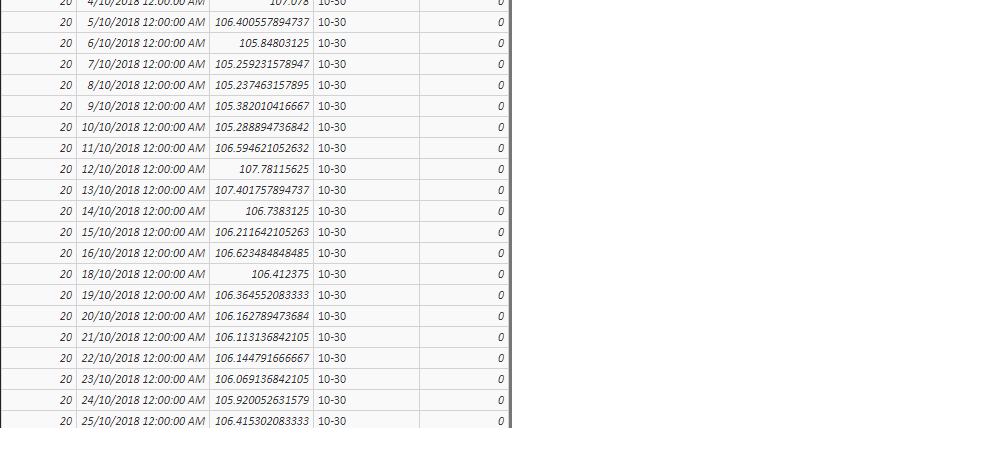cancel
Showing results for
Did you mean:Anonymous
Not applicable

## Using slicer to change dates for calculating difference column

Hi all,

I'm at a loss here and would love some help.This is what my data looks like. The depth column increases by 0.5, which is why I created the groups column. Another example of the data below.My goal is to have a graph similar to the one below, but one which shows the temperature difference based on the date input from the slicer/slider.For example if the slider is set from 7/10/2018 to 14/10/2018, the temperature difference at a depth of 20m would be given as 106.738 - 105.259 = 1.479. This calculation after being replicated for the other depths would then be plotted in their groups similar to the above picture.

Can this be done? I have tried many DAX statements to create columns but even then they are static and cannot be changed via a slicer.

Cheers,

Visura

1 ACCEPTED SOLUTIONAnonymous
Not applicable

How many data points are there for each depth and time?  Assuming only row for each combination, the following code shoud work.  Note that this is a measure, not a calculated column, so it will respond to a slicer.

```Delta =
VAR MaxDate =
MAX ( Table[Date] )
VAR MinDate =
MIN ( Table[Date] )
VAR MinTemp =
CALCULATE (
MIN ( Table[Temperature] ),
Table[Date] = MinDate
)
VAR MaxTemp =
CALCULATE (
MAX ( Table[Temperature] ),
Table[Date] = MaxDate
)
RETURN
MaxTemp - MinTemp```
2 REPLIES 2Anonymous
Not applicable

How many data points are there for each depth and time?  Assuming only row for each combination, the following code shoud work.  Note that this is a measure, not a calculated column, so it will respond to a slicer.

```Delta =
VAR MaxDate =
MAX ( Table[Date] )
VAR MinDate =
MIN ( Table[Date] )
VAR MinTemp =
CALCULATE (
MIN ( Table[Temperature] ),
Table[Date] = MinDate
)
VAR MaxTemp =
CALCULATE (
MAX ( Table[Temperature] ),
Table[Date] = MaxDate
)
RETURN
MaxTemp - MinTemp```Anonymous
Not applicable

Thanks so much! Works perfectly.

I had been struggling for hours, and didn't think that a measure could dynamically hold values like this. Big props to you Chris.

For the current data set there is only one temperature data point for each depth for a given day.  In future the frequency of the data will increase, from once/day -> once/15mins. From what I understand of this code, this approach will still work given the higher resolution, correct?